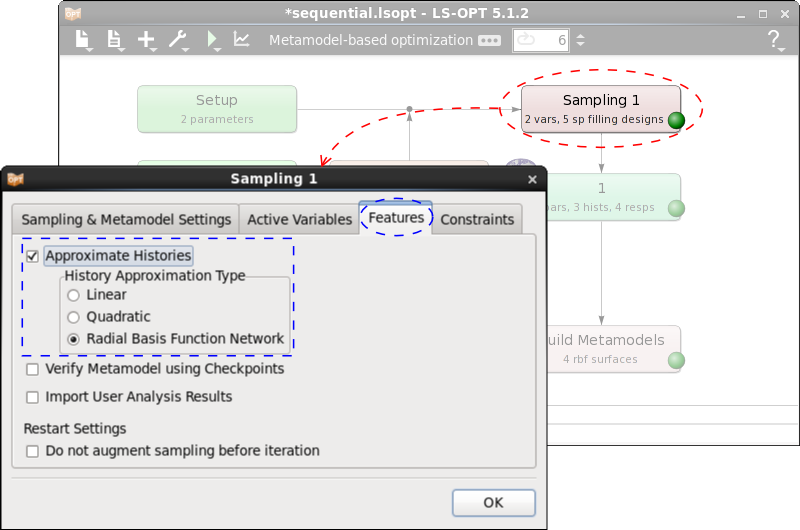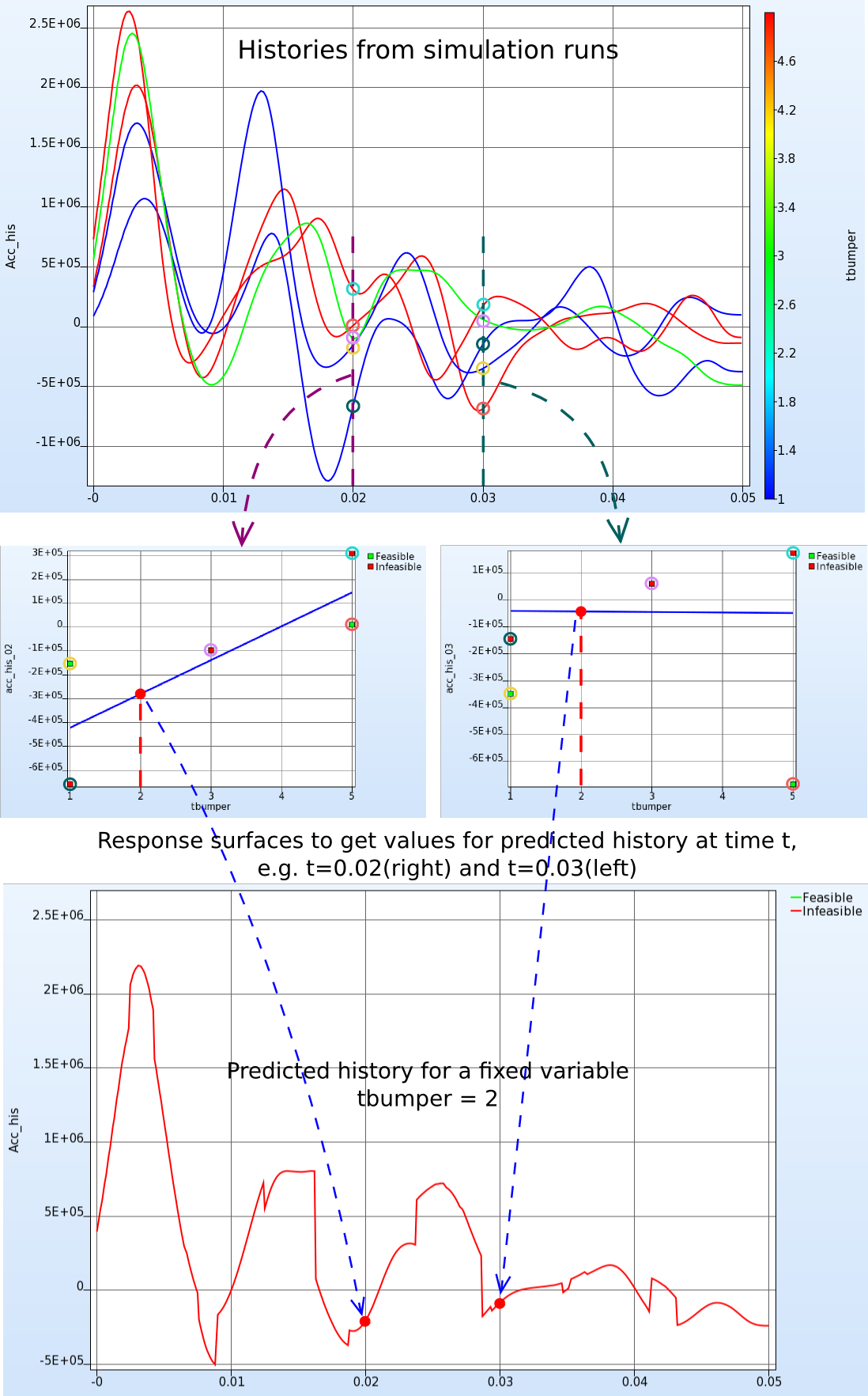x

# How are predicted histories calculated?

An extension of the metamodel concept to histories

Each history curve can be pointwise (at each sampled time-step) approximated using metamodels. These approximations of the entire history curves in time-domain are called predicted histories. These history approximations are used to study the influence of changes in the variables as well as for parameter identification problems.

The approximation of histories is enabled by setting the Approximate Histories flag on the Features page of the Sampling dialog as shown in the figure on the right.

• The user can approximate the data using either linear or quadratic polynomials or by radial basis functions.
• The approximations are carried out on the sampling points used for response approximations.While the approximation models for the histories and responses can be different, the number and location of sampling points remain the same such that all options for history approximation may not be suitable depending on the number of available data points, for example, if the response sampling is linear polynomial the number of points sampled would not be sufficient to approximate the histories using a quadratic polynomial and that option should be avoided.

It is also important to note that approximation of histories may take significantly long as approximations at thousands of time-steps are carried out.

Remarks

• It is assumed that the each history curve has the same number of time-steps for all points.
• For sequential strategies, all points sampled so far would be used for creating RBF approximations, whereas only the points sampled in the current iteration are used for polynomial approximations.

Calculation of Predicted Histories

The following steps are carried out to calculate a predicted history.

1. The simulation curves are evaluated at time = t (t = 0.02 and t = 0.03, respectively, in the figure below).
2. Those points are approximated by a metamodel of the selected type.
3. The metamodel is evaluated at the selected parameter combination (tbumper = 2 in the figure below) to get the predicted value.
4. Those steps are repeated for many equidistant time steps.
5. The predicted history is the linear interpolation of all predicted values.